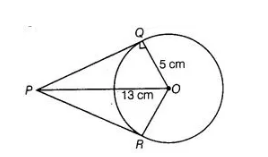# From a point P which is at a distance of 13 cm

Question:

From a point P which is at a distance of 13 cm from the centre 0 of a circle of radius 5 cm, the pair of tangents PQ and PR to the circle is drawn.

Then, the area of the quadrilateral PQOR is

(a) 60 cm2

(b) 65 cm2

(c) 30 cm2

(d) 32.5 cm2

Solution:

(a) Firstly, draw a circle of radius 5 cm having centre O. P is a point at a distance of 13 cm from O. A pair of tangents PQ and PR are drawn.$\because \quad \quad O Q \perp Q P$ [since, AP is a tangent line]

In right angled $\triangle P Q O, \quad O P^{2}=O Q^{2}+Q P^{2}$

$\Rightarrow \quad 13^{2}=5^{2}+Q P^{2}$

$\Rightarrow \quad Q P^{2}=169-25=144$

$\Rightarrow \quad Q P=12 \mathrm{~cm}$

Now, area of $\Delta O Q P=\frac{1}{2} \times Q P \times Q O$

$=\frac{1}{2} \times 12 \times 5=30 \mathrm{~cm}^{2}$

$\therefore \quad$ Area of quadrilateral $Q O R P=2 \triangle O Q P$

$=2 \times 30=60 \mathrm{~cm}^{2}$